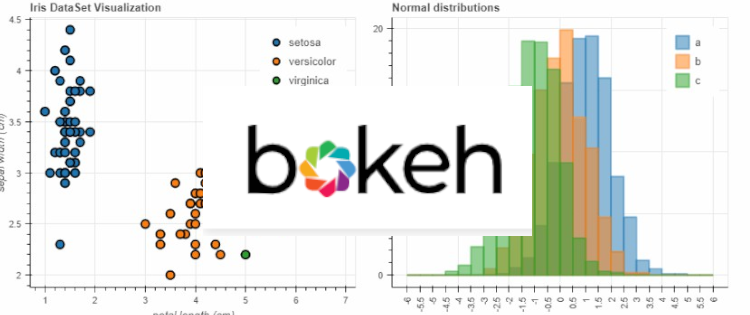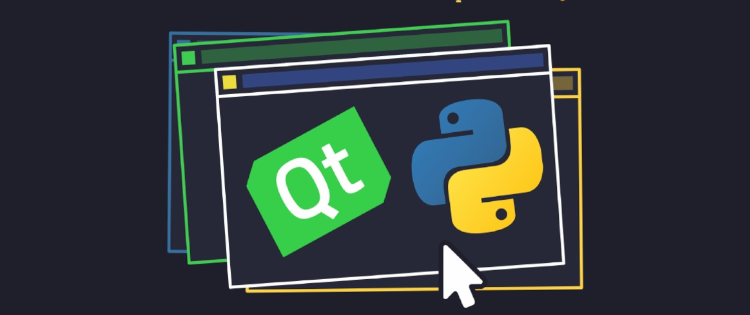# 数据可视化 – 利用Bokeh和Bottle.py在网上展示你的数据

• xhyl
• February 5, 2020

## 1. 文章重点和项目介绍

https://github.com/pythonlibrary/bokeh-bottlepy

## 2. 数据集研究和图表准备

 ``````1 2 3 4 5 6 7 8 `````` ``````import numpy as np import pandas as pd from bokeh.plotting import figure, show from bokeh.models import ColumnDataSource, HoverTool from bokeh.transform import dodge from bokeh.io import output_notebook ``````

 ``````1 `````` ``````output_notebook() ``````### 2.1 导入数据集

 ``````1 2 3 4 5 `````` ``````cities = ['上海', '北京', '杭州', '宁波', '保定', '南京', '苏州', '深圳', '厦门', '广州'] df = pd.read_csv('AQI_merged.csv') df['date'] = pd.to_datetime(df['date']) df = df.sort_values(by='date').reset_index(drop=True) df = df[df['type']=='AQI'] ``````

 ``````1 2 `````` ``````df_2019_day = df[df['date']>='2019-01-01'] df_2019_day.head() ``````### 2.2 绘制图表

• 2019年上海，北京，深圳三地的每天AQI变化曲线（曲线图）
• 2019年上海，北京，深圳三地的每月平均AQI对比（柱状图）
• 2017年到2019年北京每月平均AQI对比（柱状图）

#### 图表1：2019年上海，北京，深圳三地的每天AQI变化曲线

 `````` 1 2 3 4 5 6 7 8 9 10 11 `````` ``````source = ColumnDataSource(df_2019_day) p = figure(x_axis_type="datetime", title="2019年AQI日均平均变化曲线", plot_width=900, plot_height=400) p.line('date', '上海', line_color='blue', legend_label='上海', source=source) p.line('date', '北京', line_color='green', legend_label='北京', source=source) p.line('date', '深圳', line_color='orange', legend_label='深圳', source=source) p.legend.location = "top_right" p.add_tools(HoverTool(tooltips=[("AQI", "\$y")])) show(p) ``````#### 图表2：2019年上海，北京，深圳三地的每月平均AQI对比

 ``````1 2 3 4 `````` ``````pd.options.mode.chained_assignment = None df_2019_day['month'] = df_2019_day['date'].apply(lambda x: x.strftime('%Y-%m')) df_2019_month = df_2019_day.groupby(by='month').mean().reset_index() df_2019_month.head() ```````````` 1 2 3 4 5 6 7 8 9 10 11 12 13 14 `````` ``````source = ColumnDataSource(df_2019_month) p = figure(x_range=list(df_2019_month['month']), title="2019年AQI", plot_width=900, plot_height=400) p.vbar(x=dodge('month', -0.25, range=p.x_range), top='上海', width=0.2, color="#c9d9d3", legend_label="上海", source=source) p.vbar(x=dodge('month', 0, range=p.x_range), top='北京', width=0.2, color="#718dbf", legend_label="北京", source=source) p.vbar(x=dodge('month', 0.25, range=p.x_range), top='深圳', width=0.2, color="#e84d60", legend_label="深圳", source=source) p.xgrid.grid_line_color = None p.y_range.start = 0 p.add_tools(HoverTool(tooltips=[("时间", "@month"), ("上海平均AQI", "@{上海}"), ("北京平均AQI", "@{北京}"), ("深圳平均AQI", "@{深圳}")])) show(p) ``````#### 图表3：2017年到2019年北京每月平均AQI对比

 ``````1 2 3 4 5 `````` ``````df['date_ym'] = df['date'].apply(lambda x: x.strftime('%Y-%m')) df_month = df.groupby(by='date_ym').mean().reset_index() df_month['month'] = df_month['date_ym'].apply(lambda x: x.split('-')[-1]) df_month['year'] = df_month['date_ym'].apply(lambda x: x.split('-')) df_month.head() ````````````1 2 3 `````` ``````df_2017 = df_month[df_month['year']=='2017'][['month', '北京']] df_2018 = df_month[df_month['year']=='2018'][['month', '北京']] df_2019 = df_month[df_month['year']=='2019'][['month', '北京']] ``````

 `````` 1 2 3 4 5 6 7 8 9 10 11 12 13 14 15 16 `````` ``````source_2017 = ColumnDataSource(df_2017) source_2018 = ColumnDataSource(df_2018) source_2019 = ColumnDataSource(df_2019) p = figure(x_range=list(df_2017['month']), title="2017-2019年北京AQI对比", plot_width=900, plot_height=400) p.vbar(x=dodge('month', -0.25, range=p.x_range), top='北京', width=0.2, color="#c9d9d3", legend_label="2017", source=source_2017) p.vbar(x=dodge('month', 0, range=p.x_range), top='北京', width=0.2, color="#718dbf", legend_label="2018", source=source_2018) p.vbar(x=dodge('month', 0.25, range=p.x_range), top='北京', width=0.2, color="#e84d60", legend_label="2019", source=source_2019) p.xgrid.grid_line_color = None p.y_range.start = 0 p.add_tools(HoverTool(tooltips=[("时间", "@month"), ("AQI", "@{北京}")])) show(p) ``````## 3. Bottle网页应用

### 3.1 文件夹结构

• dataset文件夹：包含了数据集csv文件
• static文件夹：包含了bootstrap前端框架代码，包括css，JavaScript，以及fonts等，用于以bootstrap的主题来展现html页面
• views文件夹中：包含我们要如何展示数据的模板，本项目作为入门项目，其中仅仅包含了一个index.tpl文件，作为我们仅有的一个单页面网页的模板，该模板会由bottle应用导入数据来渲染，最总形成用户看到的页面
• app.py：为我们的入口文件，我们所有的python代码将在这个里边实现，最终运行也是通过：python app.py来启动服务
• Procfile：涉及到Heroku部署，后边我们会提到### 3.2 路由

 `````` 1 2 3 4 5 6 7 8 9 10 11 12 13 14 15 16 17 18 `````` ``````dirname = '.' app = Bottle() debug(True) @app.route('/static/<filename:re:.*\.css>') def send_css(filename): return static_file(filename, root=dirname+'/static/asset/css') @app.route('/static/<filename:re:.*\.js>') def send_js(filename): return static_file(filename, root=dirname+'/static/asset/js') @app.route('/') def index(): data = { "developer_organization":"pythonlibrary.net"} return template('index', data = data) ``````

### 3.3 模板实现

 ``````1 2 3 `````` `````` China AQI ``````

 ``````1 2 3 4 5 6 `````` `````` ``````
 ``````1 2 3 4 5 6 `````` ``````

``````

 ``````1 2 3 4 5 `````` ``````@app.route('/') def index(): data = { "developer_organization":"pythonlibrary.net"} return template('index', data = data) ``````

### 3.4 启动网页服务

 ``````1 2 3 `````` ``````if __name__ == "__main__": port = 8080 app.run(host="0.0.0.0", port=port, debug=True) ``````

## 4. 将Bokeh和Bottle集成在一起

### 4.1 模板修改

 ``````1 2 3 `````` `````` ``````

 `````` 1 2 3 4 5 6 7 8 9 10 11 `````` ``````
{{!data["plot1_div"]}}

{{!data["plot2_div"]}}

{{!data["plot3_div"]}}
``````

``````{{!data["plot_script"]}}
``````

### 4.2 Python代码集成

 `````` 1 2 3 4 5 6 7 8 9 10 11 12 13 14 15 16 17 18 19 20 21 22 23 24 25 26 27 28 29 30 31 32 33 34 35 36 37 38 39 40 41 42 43 44 45 46 47 48 49 50 51 52 53 54 55 56 57 58 59 60 61 62 63 64 65 66 67 68 69 70 `````` ``````def get_df_from_source(): ''' get dataframes from the source dataset, only take the data of some big cities ''' cities = ['上海', '北京', '杭州', '宁波', '保定', '南京', '苏州', '深圳', '厦门', '广州'] df = pd.read_csv(dirname+'/dataset/AQI_merged.csv') df['date'] = pd.to_datetime(df['date']) df = df.sort_values(by='date').reset_index(drop=True) df = df[df['type']=='AQI'] return df def draw_daily_AQI(mini_date, df): year = mini_date.split('-') df_day = df[df['date']>=mini_date] source = ColumnDataSource(df_day) p = figure(x_axis_type="datetime", title="{}年AQI日均平均变化曲线".format(year), plot_width=1150, plot_height=400) p.line('date', '上海', line_color='blue', legend_label='上海', source=source) p.line('date', '北京', line_color='green', legend_label='北京', source=source) p.line('date', '深圳', line_color='orange', legend_label='深圳', source=source) p.legend.location = "top_right" p.add_tools(HoverTool(tooltips=[("AQI", "\$y")])) return p def draw_month_AQI(mini_date, df): year = mini_date.split('-') df_day = df[df['date']>=mini_date] df_day['month'] = df_day['date'].apply(lambda x: x.strftime('%Y-%m')) df_month = df_day.groupby(by='month').mean().reset_index() source = ColumnDataSource(df_month) p = figure(x_range=list(df_month['month']), title="2019年AQI", plot_width=1150, plot_height=400) p.vbar(x=dodge('month', -0.25, range=p.x_range), top='上海', width=0.2, color="#c9d9d3", legend_label="上海", source=source) p.vbar(x=dodge('month', 0, range=p.x_range), top='北京', width=0.2, color="#718dbf", legend_label="北京", source=source) p.vbar(x=dodge('month', 0.25, range=p.x_range), top='深圳', width=0.2, color="#e84d60", legend_label="深圳", source=source) p.xgrid.grid_line_color = None p.y_range.start = 0 p.add_tools(HoverTool(tooltips=[("时间", "@month"), ("上海平均AQI", "@{上海}"), ("北京平均AQI", "@{北京}"), ("深圳平均AQI", "@{深圳}")])) return p def draw_year_AQI(df): df['date_ym'] = df['date'].apply(lambda x: x.strftime('%Y-%m')) df_month = df.groupby(by='date_ym').mean().reset_index() df_month['month'] = df_month['date_ym'].apply(lambda x: x.split('-')[-1]) df_month['year'] = df_month['date_ym'].apply(lambda x: x.split('-')) df_2017 = df_month[df_month['year']=='2017'][['month', '北京']] df_2018 = df_month[df_month['year']=='2018'][['month', '北京']] df_2019 = df_month[df_month['year']=='2019'][['month', '北京']] source_2017 = ColumnDataSource(df_2017) source_2018 = ColumnDataSource(df_2018) source_2019 = ColumnDataSource(df_2019) p = figure(x_range=list(df_2017['month']), title="2017-2019年北京AQI对比", plot_width=1150, plot_height=400) p.vbar(x=dodge('month', -0.25, range=p.x_range), top='北京', width=0.2, color="#c9d9d3", legend_label="2017", source=source_2017) p.vbar(x=dodge('month', 0, range=p.x_range), top='北京', width=0.2, color="#718dbf", legend_label="2018", source=source_2018) p.vbar(x=dodge('month', 0.25, range=p.x_range), top='北京', width=0.2, color="#e84d60", legend_label="2019", source=source_2019) p.xgrid.grid_line_color = None p.y_range.start = 0 p.add_tools(HoverTool(tooltips=[("时间", "@month"), ("AQI", "@{北京}")])) return p ``````

 `````` 1 2 3 4 5 6 7 8 9 10 11 12 13 14 15 `````` ``````@app.route('/') def index(): df = get_df_from_source() plot1 = draw_daily_AQI('2019-01-01', df=df) plot2 = draw_month_AQI('2019-01-01', df=df) plot3 = draw_year_AQI(df=df) plots_data = components((plot1, plot2, plot3)) data = { "plot_script":plots_data, "plot1_div":plots_data, "plot2_div":plots_data, "plot3_div":plots_data, "developer_organization":"pythonlibrary.net"} return template('index', data = data) ``````## 5. 部署应用到Heroku

``````web: python app.py
``````

 ``````1 2 3 `````` ``````if __name__ == "__main__": port=int(os.environ.get("PORT", 8080)) app.run(host="0.0.0.0", port=port, debug=True ``````

## 类似文章### PyQt5教程4 – 发现局域网的其他客户端

• xhyl
• January 29, 2020### PyQt5教程3 – 发送页面进度条，自定义信号槽和线程

• xhyl
• January 22, 2020### PyQt5教程2：主页面和拖动

• xhyl
• January 15, 2020